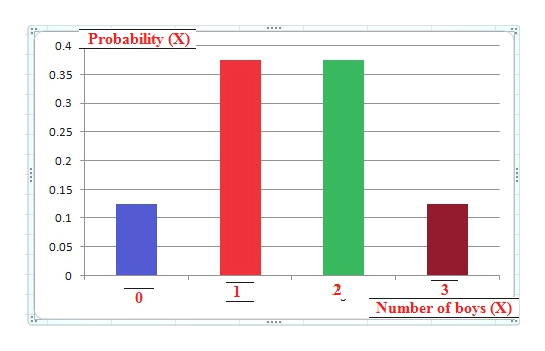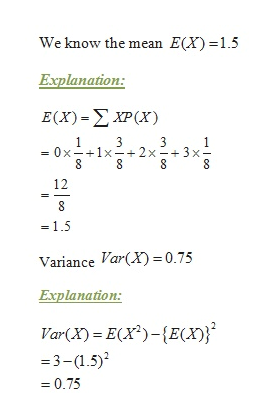# construct a probability distribution and draw a graph for a family of 3 children.  Let X represent the number of boys. Find the mean, variance, ad standard deviation for the distribution.

Question
884 views

construct a probability distribution and draw a graph for a family of 3 children.  Let X represent the number of boys. Find the mean, variance, ad standard deviation for the distribution.

check_circle

Step 1

According to the given question, construct a probability distribution and draw a graph for a family of 3 children.  Let X represents the number of boys. Therefore the x can be considering the following sample space:

 Sample Space observation X value GGG 0 GGB,BGG,GBG 1 GBB,BBG,BGB 2 BBB 3

Therefore total possible cases 8 and the corresponding probably (discrete) distribution a for a family of 3 children is as follows:

 Number of boys (X) 0 1 2 3 Total Probability (X) 1/8 3/8 3/8 1/8 1

The graph for a family of 3 children is as follows:help_outlineImage TranscriptioncloseProbability (X) 0.4 0.35 0.3 0.25 0.2 0.15 0.1 0.05 0 3 0 Number of boys (X) - fullscreen
Step 2

Mean=1.5, varaince =0.75 and standard deviation=0.866,...help_outlineImage TranscriptioncloseWe know the mean E(X) 1.5 Explanation: E(X)-ΣΧP (X) 3 0x 1x+2x-3x- 8 8 12 8 1.5 Variance Var(X = 0.75 Explanation Var(X) E(x)-E(X) -3-(1.5)2 0.75 enco nco fullscreen

### Want to see the full answer?

See Solution

#### Want to see this answer and more?

Solutions are written by subject experts who are available 24/7. Questions are typically answered within 1 hour.*

See Solution
*Response times may vary by subject and question.
Tagged in

### Data